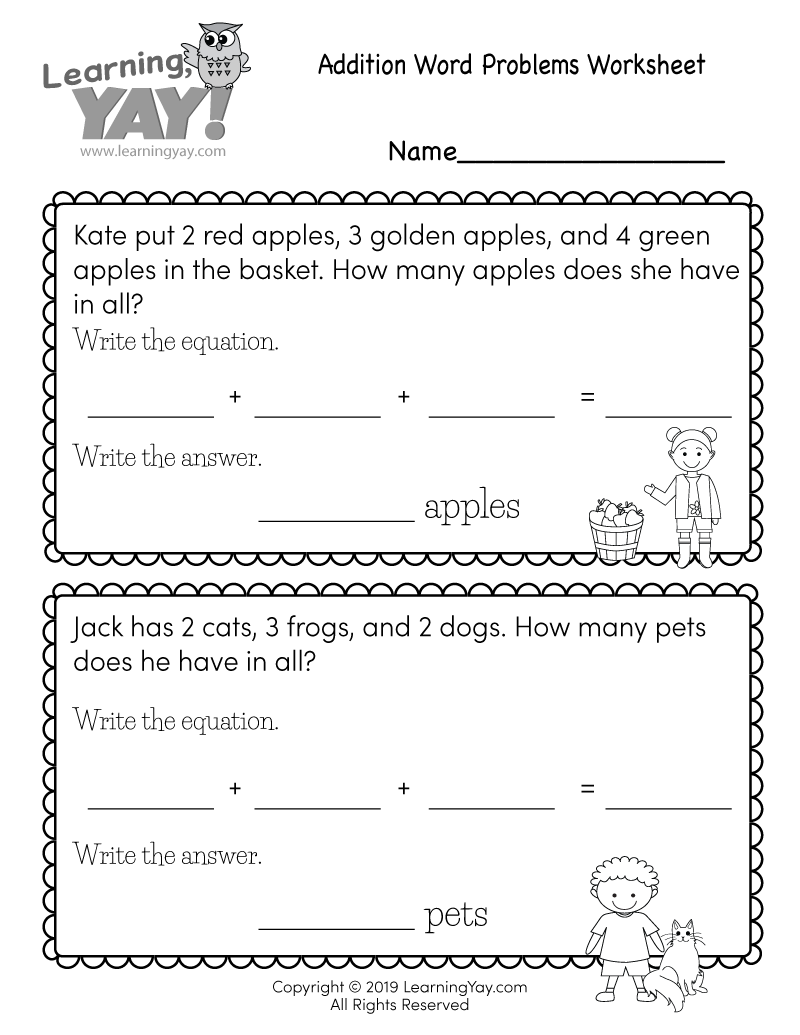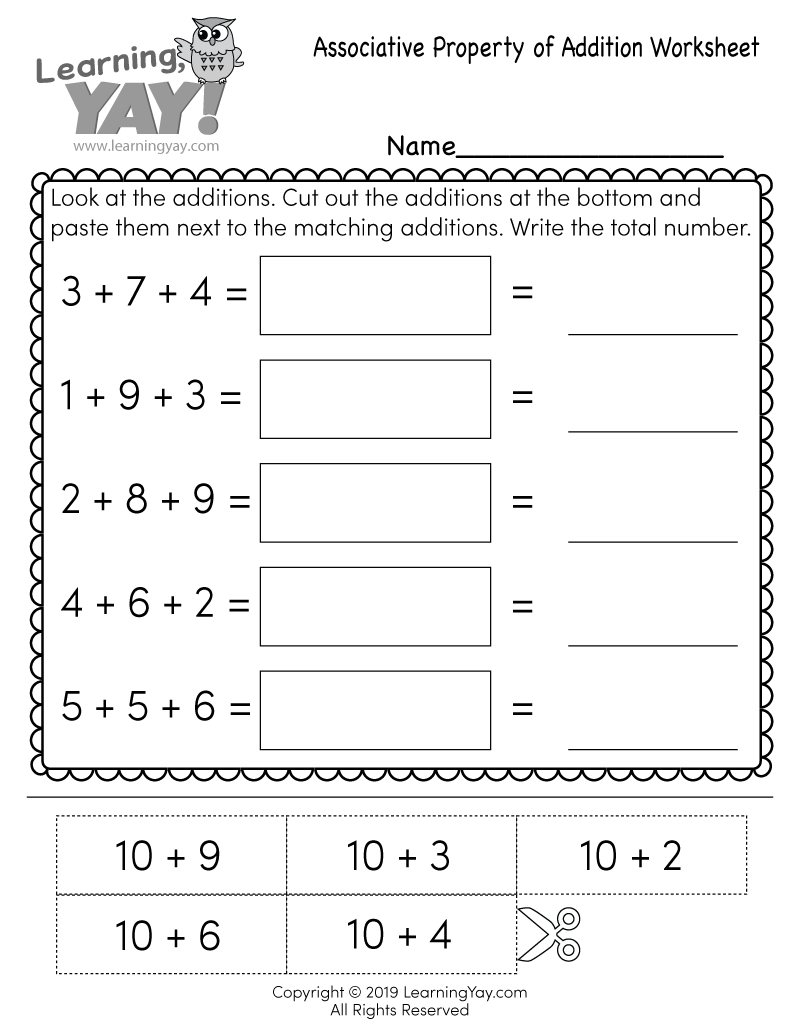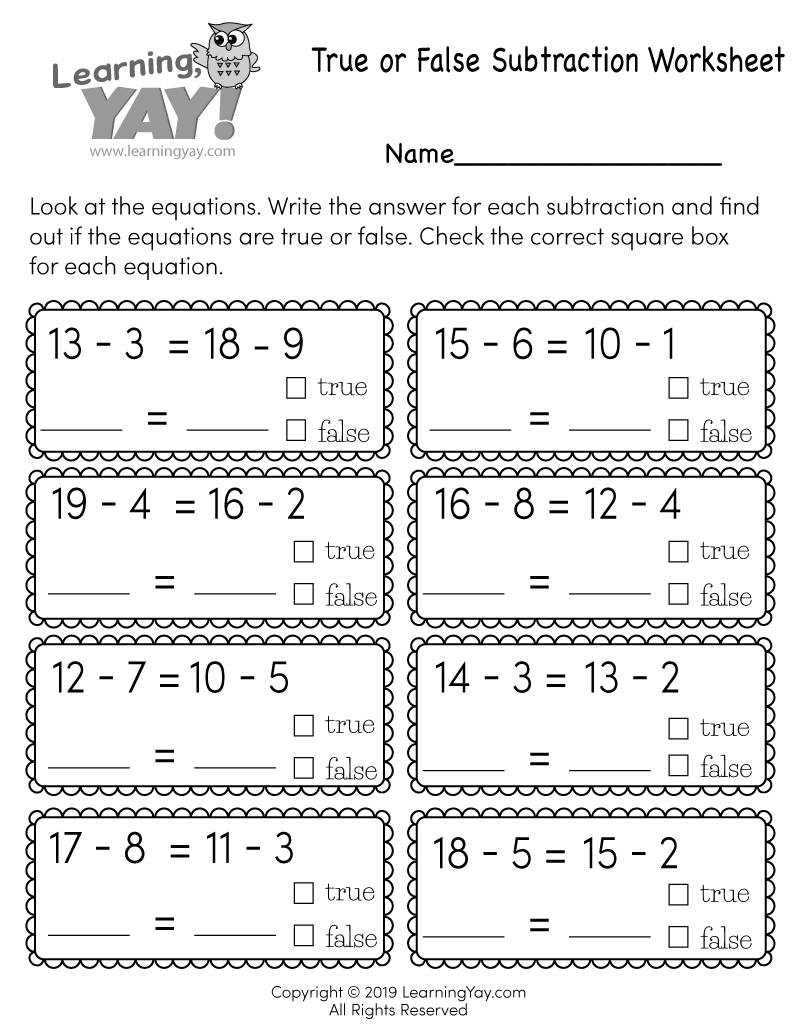# Addition Word Problems Worksheet

First graders can practice decoding addition word problems into algebraic equations with this free printable worksheet. They are asked to add three whole numbers whose sum is less than 20 in order to solve simple math problems. It’s an easy way for children to build their critical thinking skills.This free printable math worksheet is aligned to first grade Common Core standards. It is an excellent resource for teachers and parents who are teaching the following standard.
##### 1.OA.A.2 - Common Core ID

Solve word problems that call for addition of three whole numbers whose sum is less than or equal to 20, e.g., by using objects, drawings, and equations with a symbol for the unknown number to represent the problem. Understand and apply properties of operations and the relationship between addition and subtraction.

Common Core » 1st Grade Math Standards » Operations & Algebraic Thinking » Represent and solve problems involving addition and subtraction. » 1.OA.A.2

## There are multiple ways to get this addition worksheet.

### You might also like these math worksheets: